Anzeige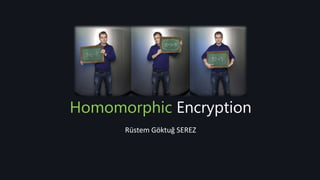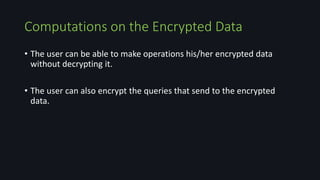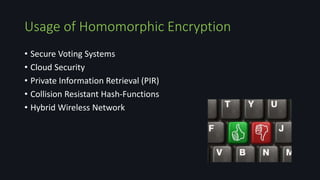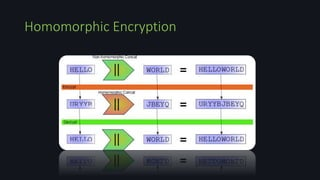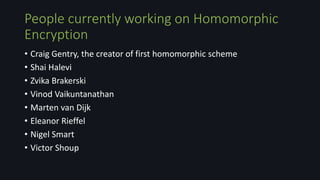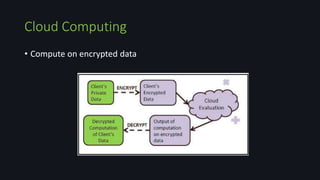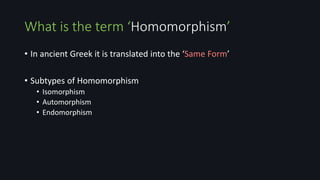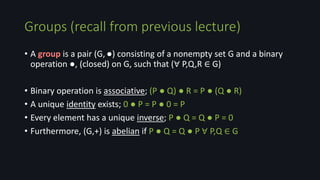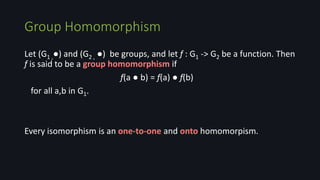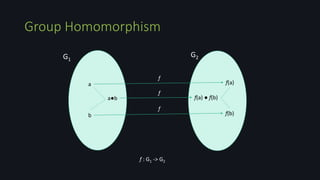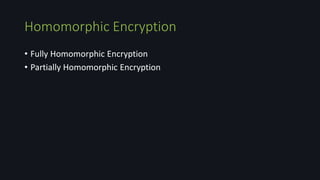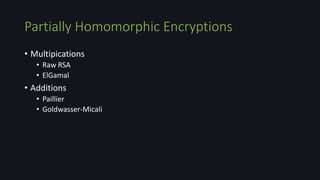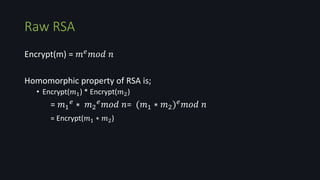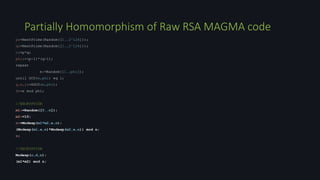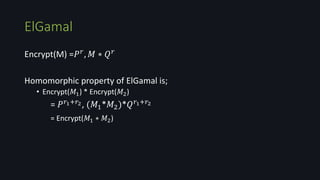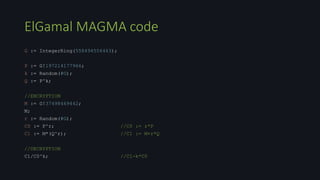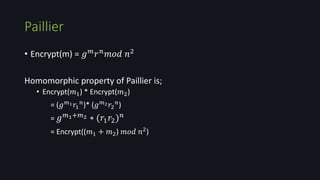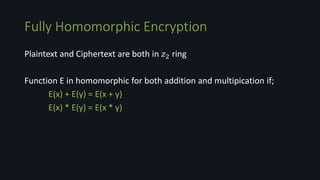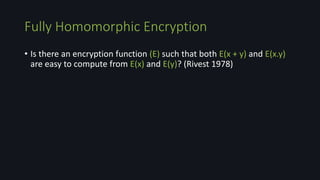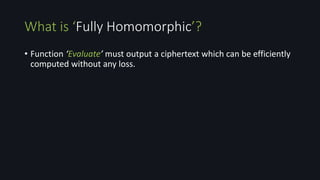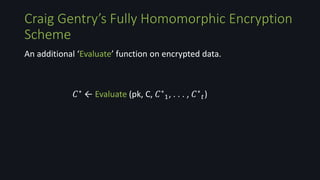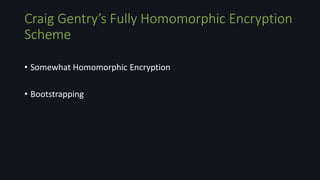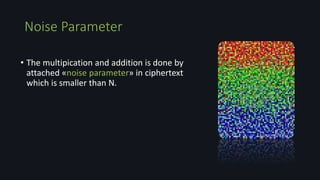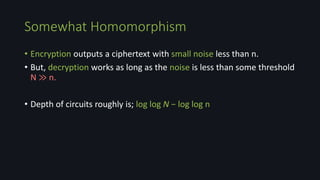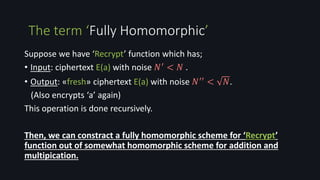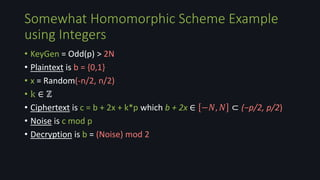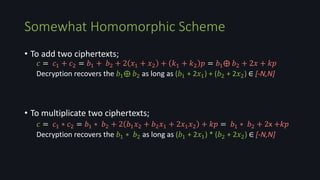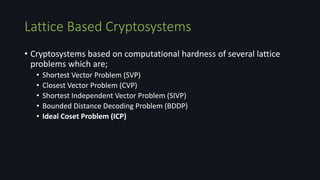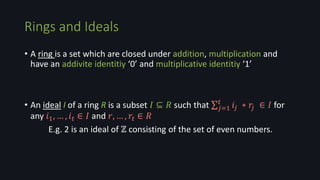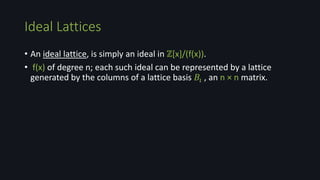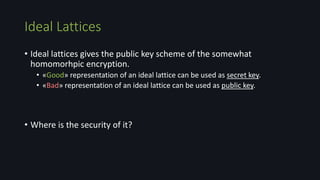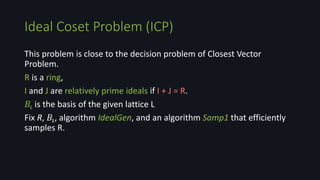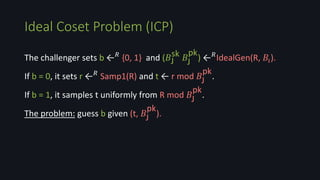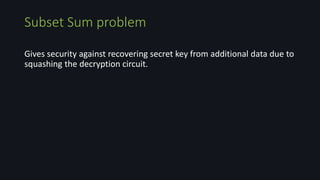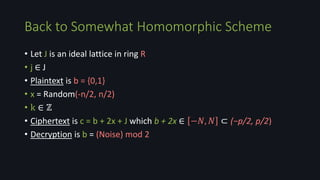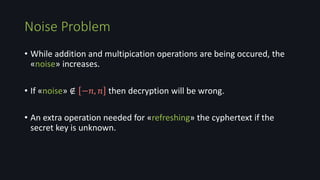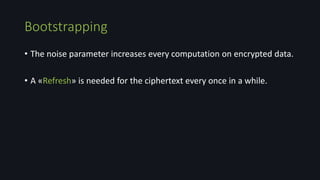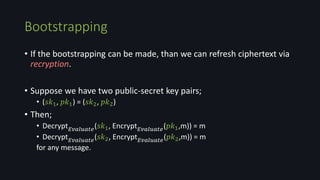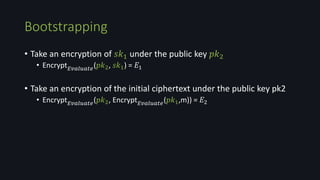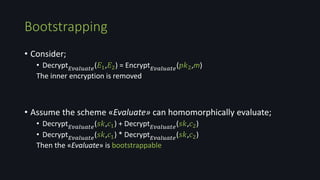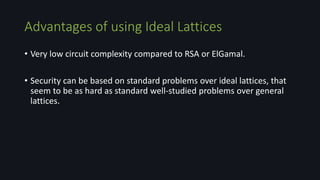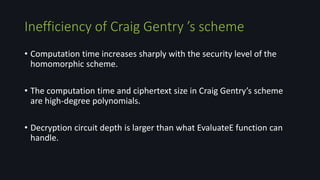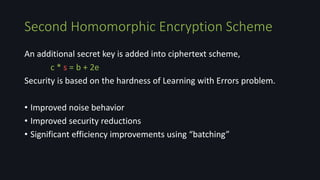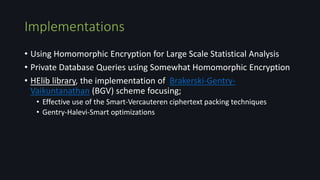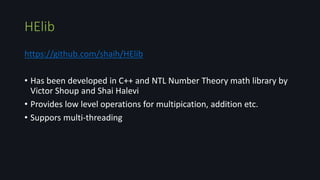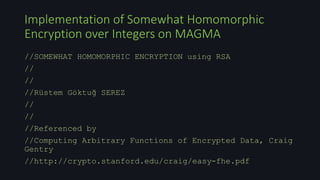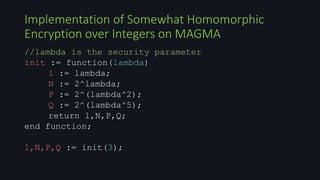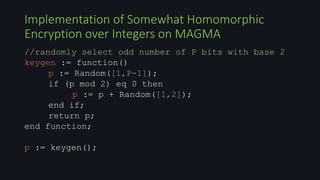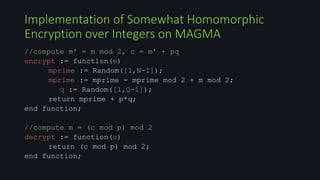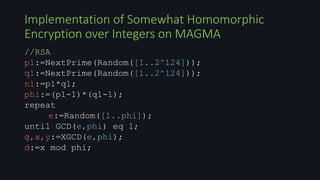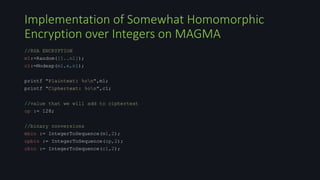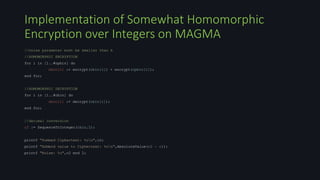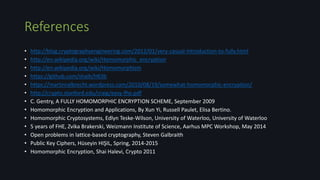1 von 58
Anzeige

### Homomorphic Encryption

1. Homomorphic Encryption Rüstem Göktuğ SEREZ
2. Computations on the Encrypted Data • The user can be able to make operations his/her encrypted data without decrypting it. • The user can also encrypt the queries that send to the encrypted data.
3. Usage of Homomorphic Encryption • Secure Voting Systems • Cloud Security • Private Information Retrieval (PIR) • Collision Resistant Hash-Functions • Hybrid Wireless Network
4. Homomorphic Encryption
5. People currently working on Homomorphic Encryption • Craig Gentry, the creator of first homomorphic scheme • Shai Halevi • Zvika Brakerski • Vinod Vaikuntanathan • Marten van Dijk • Eleanor Rieffel • Nigel Smart • Victor Shoup
6. Cloud Computing • Compute on encrypted data
7. What is the term ‘Homomorphism’ • In ancient Greek it is translated into the ‘Same Form’ • Subtypes of Homomorphism • Isomorphism • Automorphism • Endomorphism
8. Groups (recall from previous lecture) • A group is a pair (G,●) consisting of a nonempty set G and a binary operation ●, (closed) on G, such that (∀ P,Q,R ∈ G) • Binary operation is associative; (P ● Q) ● R = P ● (Q ● R) • A unique identity exists; 0 ● P = P ● 0 = P • Every element has a unique inverse; P ● Q = Q ● P = 0 • Furthermore, (G,+) is abelian if P ● Q = Q ● P ∀ P,Q ∈ G
9. Group Homomorphism Let (G1 ,●) and (G2 , ●) be groups, and let f : G1 -> G2 be a function. Then f is said to be a group homomorphism if f(a ● b) = f(a) ● f(b) for all a,b in G1. Every isomorphism is an one-to-one and onto homomorpism.
10. Group Homomorphism G2G1 a b a●b f(a) f(b) f : G1 -> G2 f(a) ● f(b) f f f
11. Homomorphic Encryption • Fully Homomorphic Encryption • Partially Homomorphic Encryption
12. Partially Homomorphic Encryptions • Multipications • Raw RSA • ElGamal • Additions • Paillier • Goldwasser-Micali
13. Raw RSA Encrypt(m) = 𝑚 𝑒 𝑚𝑜𝑑 𝑛 Homomorphic property of RSA is; • Encrypt(𝑚1) * Encrypt(𝑚2) = 𝑚1 𝑒 ∗ 𝑚2 𝑒 𝑚𝑜𝑑 𝑛= (𝑚1 ∗ 𝑚2) 𝑒 𝑚𝑜𝑑 𝑛 = Encrypt(𝑚1 ∗ 𝑚2)
14. Raw RSA MAGMA code p:=NextPrime(Random([1..2^124])); q:=NextPrime(Random([1..2^124])); n:=p*q; phi:=(p-1)*(q-1); repeat e:=Random([1..phi]); until GCD(e,phi) eq 1; g,x,y:=XGCD(e,phi); d:=x mod phi; //ENCRYPTION m:=Random([0..n]); c:=Modexp(m,e,n); m; //DECRYPTION Modexp(c,d,n);
15. Partially Homomorphism of Raw RSA MAGMA code p:=NextPrime(Random([1..2^124])); q:=NextPrime(Random([1..2^124])); n:=p*q; phi:=(p-1)*(q-1); repeat e:=Random([1..phi]); until GCD(e,phi) eq 1; g,x,y:=XGCD(e,phi); d:=x mod phi; //ENCRYPTION m1:=Random([0..n]); m2:=10; c:=Modexp(m1*m2,e,n); (Modexp(m1,e,n)*Modexp(m2,e,n)) mod n; c; //DECRYPTION Modexp(c,d,n); (m1*m2) mod n;
16. ElGamal Encrypt(M) =𝑃 𝑟, 𝑀 ∗ 𝑄 𝑟 Homomorphic property of ElGamal is; • Encrypt(𝑀1) * Encrypt(𝑀2) = 𝑃 𝑟1+𝑟2, (𝑀1*𝑀2)*𝑄 𝑟1+𝑟2 = Encrypt(𝑀1 ∗ 𝑀2)
17. ElGamal MAGMA code G := IntegerRing(558494556463); P := G!197214177966; k := Random(#G); Q := P^k; //ENCRYPTION M := G!37498469442; M; r := Random(#G); C0 := P^r; //C0 := r*P C1 := M*(Q^r); //C1 := M+r*Q //DECRYPTION C1/C0^k; //C1-k*C0
18. Partially Homomorphism of ElGamal MAGMA code G := IntegerRing(558494556463); P := G!197214177966; k := Random(#G); Q := P^k; //ENCRYPTION M1 := G!37498469442; M2 := G!48494459451; r1 := Random(#G); r2 := Random(#G); C00 := P^r1; //C00 := r1*P C10 := M1*(Q^r1); //C10 := M1+r1*Q C01 := P^r2; //C01 := r2*P C11 := M2*(Q^r2); //C11 := M2+r2*Q Ca := P^(r1+r2); //Ca := P*(r1+r2) Cb := M1*M2*(Q^(r1+r2)); //Cb := (M1 + M2)+Q*(r1+r2) C00 * C01; C10 * C11; Ca; Cb; //DECRYPTION Cb/Ca^k; M1*M2;
19. Paillier • Encrypt(m) = 𝑔 𝑚 𝑟 𝑛 𝑚𝑜𝑑 𝑛2 Homomorphic property of Paillier is; • Encrypt(𝑚1) * Encrypt(𝑚2) = (𝑔 𝑚1 𝑟1 𝑛)* (𝑔 𝑚2 𝑟2 𝑛) = 𝑔 𝑚1+𝑚2 ∗ (𝑟1 𝑟2) 𝑛 = Encrypt((𝑚1 + 𝑚2) 𝑚𝑜𝑑 𝑛2 )
20. Fully Homomorphic Encryption Plaintext and Ciphertext are both in 𝑧2 ring Function E in homomorphic for both addition and multipication if; E(x) + E(y) = E(x + y) E(x) * E(y) = E(x * y)
21. Fully Homomorphic Encryption • Is there an encryption function (E) such that both E(x + y) and E(x.y) are easy to compute from E(x) and E(y)? (Rivest 1978)
22. What is ‘Fully Homomorphic’? • Function ‘Evaluate’ must output a ciphertext which can be efficiently computed without any loss.
23. Craig Gentry’s Fully Homomorphic Encryption Scheme An additional ‘Evaluate’ function on encrypted data. 𝐶∗ ← Evaluate (pk, C, 𝐶∗ 1, . . . , 𝐶∗ 𝑡)
24. Craig Gentry’s Fully Homomorphic Encryption Scheme
25. Craig Gentry’s Fully Homomorphic Encryption Scheme • Somewhat Homomorphic Encryption • Bootstrapping
26. Noise Parameter • The multipication and addition is done by attached «noise parameter» in ciphertext which is smaller than N.
27. Somewhat Homomorphism • Encryption outputs a ciphertext with small noise less than n. • But, decryption works as long as the noise is less than some threshold N ≫ n. • Depth of circuits roughly is; log log N − log log n
28. Suppose we have ‘Recrypt’ function which has; • Input: ciphertext E(a) with noise 𝑁′ < 𝑁 . • Output: «fresh» ciphertext E(a) with noise 𝑁′′ < 𝑁. (Also encrypts ‘a’ again) This operation is done recursively. Then, we can constract a fully homomorphic scheme for ‘Recrypt’ function out of somewhat homomorphic scheme for addition and multipication. The term ‘Fully Homomorphic’
29. Somewhat Homomorphic Scheme Example using Integers • KeyGen = Odd(p) > 2N • Plaintext is b = {0,1} • x = Random(-n/2, n/2) • k ∈ ℤ • Ciphertext is c = b + 2x + k*p which b + 2x ∈ −𝑁, 𝑁 ⊂ (−p/2, p/2) • Noise is c mod p • Decryption is b = (Noise) mod 2
30. Somewhat Homomorphic Scheme • To add two ciphertexts; 𝑐 = 𝑐1 + 𝑐2 = 𝑏1 + 𝑏2 + 2 𝑥1 + 𝑥2 + 𝑘1 + 𝑘2 𝑝 = 𝑏1⨁ 𝑏2 + 2𝑥 + 𝑘𝑝 Decryption recovers the 𝑏1⨁ 𝑏2 as long as (𝑏1 + 2𝑥1) + (𝑏2 + 2𝑥2) ∈ [-N,N] • To multiplicate two ciphertexts; 𝑐 = 𝑐1 ∗ 𝑐2 = 𝑏1 ∗ 𝑏2 + 2 𝑏1 𝑥2 + 𝑏2 𝑥1 + 2𝑥1 𝑥2 + 𝑘𝑝 = 𝑏1 ∗ 𝑏2 + 2x +𝑘𝑝 Decryption recovers the 𝑏1 ∗ 𝑏2 as long as (𝑏1 + 2𝑥1) * (𝑏2 + 2𝑥2) ∈ [-N,N]
31. Lattice Based Cryptosystems • Cryptosystems based on computational hardness of several lattice problems which are; • Shortest Vector Problem (SVP) • Closest Vector Problem (CVP) • Shortest Independent Vector Problem (SIVP) • Bounded Distance Decoding Problem (BDDP) • Ideal Coset Problem (ICP)
32. Rings and Ideals • A ring is a set which are closed under addition, multiplication and have an addivite identitiy ‘0’ and multiplicative identitiy ‘1’ • An ideal I of a ring R is a subset 𝐼 ⊆ 𝑅 such that 𝑗=1 𝑡 𝑖𝑗 ∗ 𝑟𝑗 ∈ 𝐼 for any 𝑖1, … , 𝑖 𝑡 ∈ 𝐼 and 𝑟, … , 𝑟𝑡 ∈ 𝑅 E.g. 2 is an ideal of ℤ consisting of the set of even numbers.
33. • An ideal lattice, is simply an ideal in ℤ[x]/(f(x)). • f(x) of degree n; each such ideal can be represented by a lattice generated by the columns of a lattice basis 𝐵𝚤 , an n × n matrix. Ideal Lattices
34. Ideal Lattices • Ideal lattices gives the public key scheme of the somewhat homomorhpic encryption. • «Good» representation of an ideal lattice can be used as secret key. • «Bad» representation of an ideal lattice can be used as public key. • Where is the security of it?
35. Ideal Coset Problem (ICP) This problem is close to the decision problem of Closest Vector Problem. R is a ring, I and J are relatively prime ideals if I + J = R. 𝐵𝚤 is the basis of the given lattice L Fix R, 𝐵𝚤, algorithm IdealGen, and an algorithm Samp1 that efficiently samples R.
36. The challenger sets b ← 𝑅 {0, 1} and (𝐵J sk 𝐵J pk ) ← 𝑅 IdealGen(R, 𝐵𝚤). If b = 0, it sets r ← 𝑅 Samp1(R) and t ← r mod 𝐵J pk . If b = 1, it samples t uniformly from R mod 𝐵J pk . The problem: guess b given (t, 𝐵J pk ). Ideal Coset Problem (ICP)
37. Subset Sum problem Gives security against recovering secret key from additional data due to squashing the decryption circuit.
38. • Let J is an ideal lattice in ring R • j ∈ J • Plaintext is b = {0,1} • x = Random(-n/2, n/2) • k ∈ ℤ • Ciphertext is c = b + 2x + J which b + 2x ∈ −𝑁, 𝑁 ⊂ (−p/2, p/2) • Decryption is b = (Noise) mod 2 Back to Somewhat Homomorphic Scheme
39. Noise Problem • While addition and multipication operations are being occured, the «noise» increases. • If «noise» ∉ −𝑛, 𝑛 then decryption will be wrong. • An extra operation needed for «refreshing» the cyphertext if the secret key is unknown.
40. • A self-sustaining process without requiring any external help. Bootstrapping
41. Bootstrapping • The noise parameter increases every computation on encrypted data. • A «Refresh» is needed for the ciphertext every once in a while.
42. • If the bootstrapping can be made, than we can refresh ciphertext via recryption. • Suppose we have two public-secret key pairs; • (𝑠𝑘1, 𝑝𝑘1) = (𝑠𝑘2, 𝑝𝑘2) • Then; • Decrypt 𝐸𝑣𝑎𝑙𝑢𝑎𝑡𝑒(𝑠𝑘1, Encrypt 𝐸𝑣𝑎𝑙𝑢𝑎𝑡𝑒(𝑝𝑘1,m)) = m • Decrypt 𝐸𝑣𝑎𝑙𝑢𝑎𝑡𝑒(𝑠𝑘2, Encrypt 𝐸𝑣𝑎𝑙𝑢𝑎𝑡𝑒(𝑝𝑘2,m)) = m for any message. Bootstrapping
43. • Take an encryption of 𝑠𝑘1 under the public key 𝑝𝑘2 • Encrypt 𝐸𝑣𝑎𝑙𝑢𝑎𝑡𝑒(𝑝𝑘2, 𝑠𝑘1) = 𝐸1 • Take an encryption of the initial ciphertext under the public key pk2 • Encrypt 𝐸𝑣𝑎𝑙𝑢𝑎𝑡𝑒(𝑝𝑘2, Encrypt 𝐸𝑣𝑎𝑙𝑢𝑎𝑡𝑒(𝑝𝑘1,m)) = 𝐸2 Bootstrapping
44. • Consider; • Decrypt 𝐸𝑣𝑎𝑙𝑢𝑎𝑡𝑒(𝐸1,𝐸2) = Encrypt 𝐸𝑣𝑎𝑙𝑢𝑎𝑡𝑒(𝑝𝑘2,m) The inner encryption is removed • Assume the scheme «Evaluate» can homomorphically evaluate; • Decrypt 𝐸𝑣𝑎𝑙𝑢𝑎𝑡𝑒(𝑠𝑘,𝑐1) + Decrypt 𝐸𝑣𝑎𝑙𝑢𝑎𝑡𝑒(𝑠𝑘,𝑐2) • Decrypt 𝐸𝑣𝑎𝑙𝑢𝑎𝑡𝑒(𝑠𝑘,𝑐1) * Decrypt 𝐸𝑣𝑎𝑙𝑢𝑎𝑡𝑒(𝑠𝑘,𝑐2) Then the «Evaluate» is bootstrappable Bootstrapping
45. Advantages of using Ideal Lattices • Very low circuit complexity compared to RSA or ElGamal. • Security can be based on standard problems over ideal lattices, that seem to be as hard as standard well-studied problems over general lattices.
46. Inefficiency of Craig Gentry ’s scheme • Computation time increases sharply with the security level of the homomorphic scheme. • The computation time and ciphertext size in Craig Gentry’s scheme are high-degree polynomials. • Decryption circuit depth is larger than what EvaluateE function can handle.
47. Second Homomorphic Encryption Scheme An additional secret key is added into ciphertext scheme, c * s = b + 2e Security is based on the hardness of Learning with Errors problem. • Improved noise behavior • Improved security reductions • Significant efficiency improvements using “batching”
48. Implementations • Using Homomorphic Encryption for Large Scale Statistical Analysis • Private Database Queries using Somewhat Homomorphic Encryption • HElib library, the implementation of Brakerski-Gentry- Vaikuntanathan (BGV) scheme focusing; • Effective use of the Smart-Vercauteren ciphertext packing techniques • Gentry-Halevi-Smart optimizations
49. HElib https://github.com/shaih/HElib • Has been developed in C++ and NTL Number Theory math library by Victor Shoup and Shai Halevi • Provides low level operations for multipication, addition etc. • Suppors multi-threading
50. Implementation of Somewhat Homomorphic Encryption over Integers on MAGMA //SOMEWHAT HOMOMORPHIC ENCRYPTION using RSA // // //Rüstem Göktuğ SEREZ // // //Referenced by //Computing Arbitrary Functions of Encrypted Data, Craig Gentry //http://crypto.stanford.edu/craig/easy-fhe.pdf
51. //lambda is the security parameter init := function(lambda) l := lambda; N := 2^lambda; P := 2^(lambda^2); Q := 2^(lambda^5); return l,N,P,Q; end function; l,N,P,Q := init(3); Implementation of Somewhat Homomorphic Encryption over Integers on MAGMA
52. //randomly select odd number of P bits with base 2 keygen := function() p := Random([1,P-1]); if (p mod 2) eq 0 then p := p + Random([1,2]); end if; return p; end function; p := keygen(); Implementation of Somewhat Homomorphic Encryption over Integers on MAGMA
53. //compute m' = m mod 2, c = m' + pq encrypt := function(m) mprime := Random([1,N-1]); mprime := mprime - mprime mod 2 + m mod 2; q := Random([1,Q-1]); return mprime + p*q; end function; //compute m = (c mod p) mod 2 decrypt := function(c) return (c mod p) mod 2; end function; Implementation of Somewhat Homomorphic Encryption over Integers on MAGMA
54. //RSA p1:=NextPrime(Random([1..2^124])); q1:=NextPrime(Random([1..2^124])); n1:=p1*q1; phi:=(p1-1)*(q1-1); repeat e:=Random([1..phi]); until GCD(e,phi) eq 1; g,x,y:=XGCD(e,phi); d:=x mod phi; Implementation of Somewhat Homomorphic Encryption over Integers on MAGMA
55. //RSA ENCRYPTION m1:=Random([1..n1]); c1:=Modexp(m1,e,n1); printf "Plaintext: %on",m1; printf "Ciphertext: %on",c1; //value that we will add to ciphertext op := 128; //binary conversions mbin := IntegerToSequence(m1,2); opbin := IntegerToSequence(op,2); cbin := IntegerToSequence(c1,2); Implementation of Somewhat Homomorphic Encryption over Integers on MAGMA
56. //noise parameter must be smaller than b //HOMOMORPHIC ENCRYPTION for i in [1..#opbin] do cbin[i] := encrypt(cbin[i]) + encrypt(opbin[i]); end for; //HOMOMORPHIC DECRYPTION for i in [1..#cbin] do cbin[i] := decrypt(cbin[i]); end for; //decimal conversion c2 := SequenceToInteger(cbin,2); printf "Summed Ciphertext: %on",c2; printf "Addend value to Ciphertext: %on",AbsoluteValue(c2 - c1); printf "Noise: %o",c2 mod 2; Implementation of Somewhat Homomorphic Encryption over Integers on MAGMA
57. References • http://blog.cryptographyengineering.com/2012/01/very-casual-introduction-to-fully.html • http://en.wikipedia.org/wiki/Homomorphic_encryption • http://en.wikipedia.org/wiki/Homomorphism • https://github.com/shaih/HElib • https://martinralbrecht.wordpress.com/2010/08/19/somewhat-homomorphic-encryption/ • http://crypto.stanford.edu/craig/easy-fhe.pdf • C. Gentry, A FULLY HOMOMORPHIC ENCRYPTION SCHEME, September 2009 • Homomorphic Encryption and Applications, By Xun Yi, Russell Paulet, Elisa Bertino. • Homomorphic Cryptosystems, Edlyn Teske-Wilson, University of Waterloo, University of Waterloo • 5 years of FHE, Zvika Brakerski, Weizmann Institute of Science, Aarhus MPC Workshop, May 2014 • Open problems in lattice-based cryptography, Steven Galbraith • Public Key Ciphers, Hüseyin HIŞIL, Spring, 2014-2015 • Homomorphic Encryption, Shai Halevi, Crypto 2011
58. Thank you for listening
Anzeige